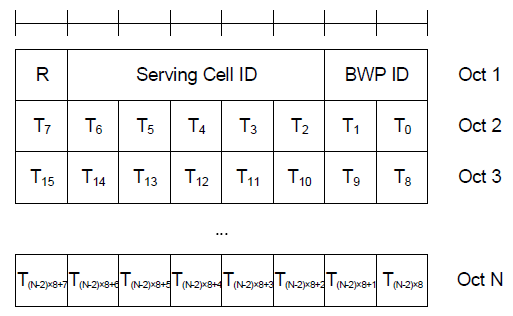5G/NR - MAC CE                                                                                Home : www.sharetechnote.com MAC CE - TCI States Activation/Deactivation for UE-specific PDSCH MAC CE   This is for extracting a subset of TCI states defined in PDSCH-Config and activating / deactivating specific TCI States. The list (a subset) of TCI states to activate/deactivate is configured by the bitmap as shown below. If a bit in a specific location is set to be '1', it means that it activate the TCI state mapped to the position of the bit. If the bit is set to be '0', it means that it deactivates the TCI state mapped to the position of the bit. For example, if T4 = 1, it activate the index 4 of tci-StatesToAddModList configured in PDSCH-Config.   < 38.321-Figure 6.1.3.14-1: TCI States Activation/Deactivation for UE-specific PDSCH MAC CE >The list of bit position that are set to be '1' is assigned to a small table called codepoint and the max size of the codepoint is 8. It mean that only up to 8 bit fields in this MAC CE can be set to be '1'. The position of '1' bits are assigned to codepoint in increasing order. For example, let's assume that following fields are set to be '1' and all other bits are set to be '0'. T4 = 1 T10 = 1 T11 = 1 T19 = 1 T25 = 1 T40 = 1 T45 = 1 T50 = 1 Then the codepoint is set to be as follows. codepoint 0 = 4 codepoint 1 = 10 codepoint 2 = 11 codepoint 3 = 19 codepoint 4 = 25 codepoint 5 = 40 codepoint 6 = 45 codepoint 7 = 50   The TCI(Transmission Configuration Index) in DCI 1_1 indicates this codepoint index. See here for further details.       Reference